# SAT II Physics : Momentum and Impulse

## Example Questions

### Example Question #8 : Sat Subject Test In Physics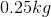ball hits a brick wall with a velocity of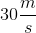and bounces back at the same speed. If the ball is in contact with the wall for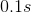, what is the value of the force exerted by the wall on the ball?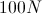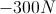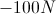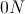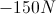Explanation:

The fastest way to solve a problem like this is with momentum.

Remember that momentum is equal to mass times velocity: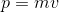. We can rewrite this equation in terms of force.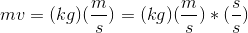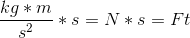Using this transformation, we can see that momentum is also equal to force times time.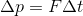can also be thought of as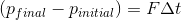.

Expand this equation to include our given values.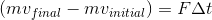Even though the ball is bouncing back at the same "speed" its velocity will now be negative, as it is moving in the opposite direction. Using this understanding we can solve for the force in our equation.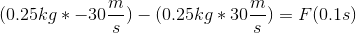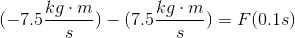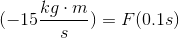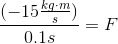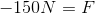Our answer is negative because the force is moving the ball in the OPPOSITE direction from the way it was originally heading.

### All SAT II Physics Resources